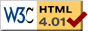### Journée-séminaire de combinatoire

#### (équipe CALIN du LIPN, université Paris-Nord, Villetaneuse)

Le 28 janvier 2020 à 10h30 en A108, Gérard Duchamp nous parlera de : One-parameter groups and combinatorial physics

Résumé : In this talk, we consider the normal ordering of operators of the type $$\Omega=\sum_{\alpha+\beta=r}c_{\alpha,\beta}(a^+)^\alpha a(a^+)^\beta,\ \ \alpha,\beta,r\textrm{ integers}$$ where $a$ (resp. $a^{+}$) is a boson annihilation (resp. creation) operator; these satisfy $[a,a^{+}]\equiv a a^{+}-a^{+}a=1$, and for the purposes of this presentation may be thought of as $a\equiv d/dx$ and $a^{+}\equiv x$. We discuss the integration of the one-parameter groups $e^{\lambda\Omega}$ and their combinatorial by-products. In particular we show how these groups can be realized as groups of substitutions with prefactor functions. To end with, we provide a recent application of the concept one-parameter groups to arithmetics.

 Dernière modification : Monday 24 January 2022Contact pour cette page : Cyril.Banderier at lipn.univ-paris13.fr